# Sorting on a Linear Array, Algorithm Assessment, Simulating Large Arrays

Tom Kelliher, CS 315

Jan. 27, 1999

### From Last Time

Loop invariants, linear arrays, sorting on linear arrays.

### Outline

1. Sorting on linear arrays, continued.

2. Parallel algorithm assessment.

3. Simulating large arrays on small arrays.

### Coming Up

Bit model, lower bounds.

# Sorting on a Linear Array

## Linear Arrays

A linear array: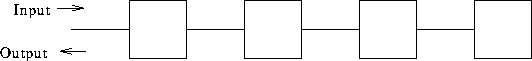Cell properties:

1. Operation sequence:

3. Perform computation indicated by local program.

4. Generate output for neighbors.

5. Update local storage.

Communication properties:

1. Data pulsing through network: systolic computation.

## Sorting

1. Phase 1: sorting program.
1. Accept input from left neighbor.

2. Compare to stored value.

3. Output larger value to right neighbor.

4. Store smaller value.

2. Phase 2: output. Begin passing left when no more input is received from the left neighbor.

Example: sort 12, 19, 6, 15.

How many steps to sort? How many steps to output?

# Algorithm Assessment

How can we measure a parallel algorithm? Apply following to sorting on the linear array.

1. Run time: T.

2. Number of processors: N.

3. Speedup: Let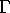be the running time of the fastest sequential algorithm. Then: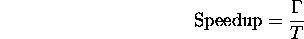What is linear speedup?

Say you have P processors. Speedup is at most P. Why? (Hint: a parallel algorithm that runs in T steps on P processors can be simulated on a sequential machine in how many steps?)

4. Work: W = TP.

Measures algorithm inefficiencies due to idle processors.

5. Efficiency of processor utilization: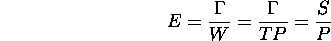6. Is it always possible to minimize T and E?

Example: consider two algorithms for solving a problem of size M:

1. M steps on an M processor machine.

2.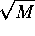steps on an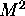processor machine.

If we have anprocessor machine and need to run the algorithm X times, which algorithm should we use? Assume theprocessor machine may be split in M M processor machines.

(Recall: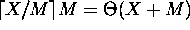.)

# Simulating a Large Array of Processors with a Small Array of Processors

Let's say we want to solve a problem of size N with P processors.

1. We generally assume that P = N. Is this valid? --- More later.

2. Suppose, we have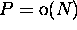fine-grain processors in a linear array. Can we sort N numbers?

Fine-grain vs. coarse-grain processing.

3. Assuming appropriate granularity, here's how we simulate 12 processors on 3 processors: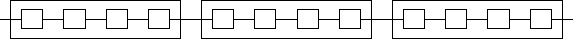What about simulating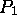processors on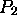processors, where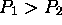?

What slowdown do we encounter?

4. Is the scaled-down algorithm less efficient?

5. So, why do we assume, in general, that P = N?

Implications:

1. Given an algorithm efficient for large P, we can construct one efficient for small P.